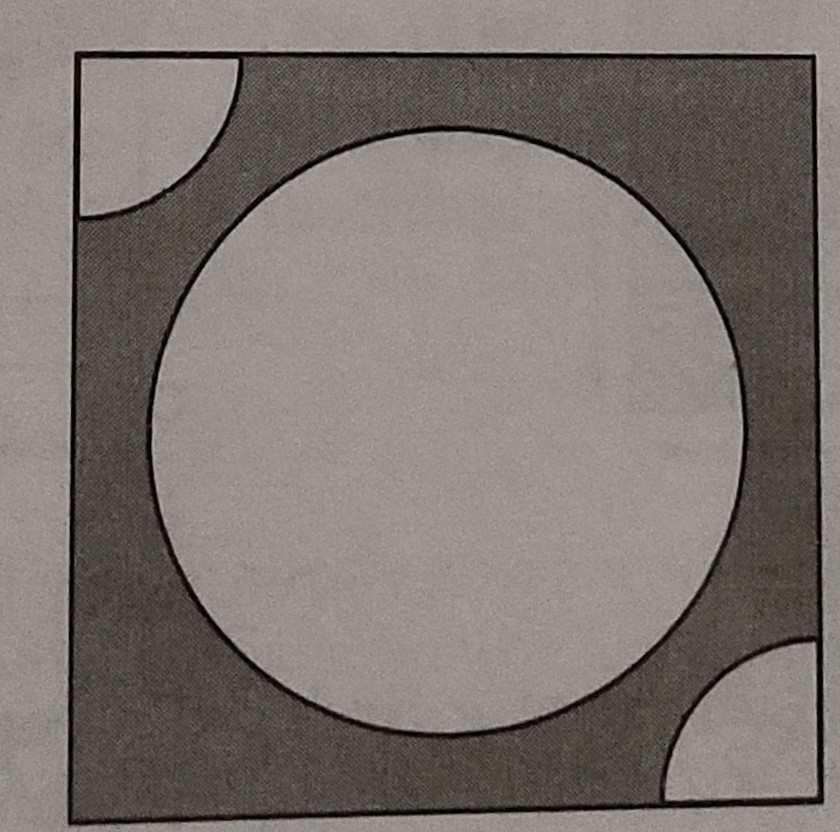"
">

# From each of the two opposite corners of a square of side $8 \mathrm{~cm}$, a quadrant of a circle of radius $1.4 \mathrm{~cm}$ is cut. Another circle of radius $4.2 \mathrm{~cm}$ is also cut from the centre as shown in the figure. Find the area of the remaining (shaded) portion of the square. $($ Use $\pi=22 / 7)$."

Given:

From each of the two opposite corners of a square of side $8 \mathrm{~cm}$, a quadrant of a circle of radius $1.4 \mathrm{~cm}$ is cut. Another circle of radius $4.2 \mathrm{~cm}$ is also cut from the centre as shown in the figure.

To do:

We have to find the area of the remaining (shaded) portion of the square.

Solution:

Length of the side of the square $ABCD = 8\ cm$

This implies,

Area of the square $=(8)^{2}$

$=64 \mathrm{~cm}^{2}$

Radius of each quadrant $=1.4 \mathrm{~cm}$

This implies,

Area of two quadrants $=2 \times \frac{1}{4} \pi r^{2}$

$=\frac{1}{2} \times \frac{22}{7}(1.4)^{2} \mathrm{~cm}^{2}$

$=\frac{1}{2} \times \frac{22}{7} \times 1.4 \times 1.4$

$=3.08 \mathrm{~cm}^{2}$

Diameter of the circle $=4.2 \mathrm{~cm}$

This implies,

Radius of the circle $=\frac{4.2}{2}$

$=2.1 \mathrm{~cm}$

Area of the circle $=\pi r^{2}$

$=\frac{22}{7}(2.1)^{2} \mathrm{~cm}^{2}$

$=\frac{22}{7} \times 2.1 \times 2.1$

$=13.86 \mathrm{~cm}^{2}$

Therefore,

Area of the shaded portion $=64-(3.08+13.86) \mathrm{cm}^{2}$

$=64-16.94$

$=47.06 \mathrm{~cm}^{2}$

The area of the remaining (shaded) portion of the square is $47.06\ cm^2$.

Updated on: 10-Oct-2022

22 Views